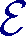coauthors Klaus Scheicher Jörg M. Thuswaldner Christiaan van de Woestijne language English published in International Journal of Number Theory 10, No. 6 (2014) pages 1459 to 1483 DOI 10.1142/S1793042114500389 supported by FWF, project S9610 (NFN S9600)

### Abstract

Letbe a commutative ring with identity and P[x] be a polynomial. In the present paper we consider digit representations in the residue class ring[x]/(P). In particular, we are interested in the question whether each A[x]/(P) can be represented modulo P in the form e0+e1X++ehXh, where the ei[x]/(P) are taken from a fixed finite set of digits. This general concept generalises both canonical number systems and digit systems over finite fields. Due to the fact that we do not assume that 0 is an element of the digit set and that P need not be monic, several new phenomena occur in this context.

### References

1. S. Akiyama, T. Borbély, H. Brunotte, A. Pethő, J.M. Thuswaldner, Generalized radix representations and dynamical systems I, Acta Math. Hungar. 108 (2005), 207—238.
2. S. Akiyama, H. Brunotte, A. Pethő, J.M. Thuswaldner, Generalized radix representations and dynamical systems II, Acta Arith. 121 (2006), 21—61.
3. S. Akiyama, C. Frougny, J. Sakarovitch, Powers of rationals modulo 1 and rational base number systems, Israel J. Math. 168 (2008), 53—91.
4. S. Akiyama, H. Rao, New criteria for canonical number systems, Acta Arith. 111 (2004), 5—25.
5. S. Akiyama, K. Scheicher, Symmetric shift radix systems and finite expansions, Math. Pannon. 18 (2007), 101—124.
6. J.-P. Allouche, E. Cateland, W.J. Gilbert, H.-O. Peitgen, J.O. Shallit, G. Skordev, Automatic maps in exotic numeration systems, Theory Comput. Syst. 30 (1997), 285—331.
7. G. Barat, V. Berthé, P. Liardet, J. Thuswaldner, Dynamical directions in numeration, Ann. Inst. Fourier (Grenoble) 56 (2006), 1987—2092.
8. T. Beck, H. Brunotte, K. Scheicher, J.M. Thuswaldner, Number systems and tilings over Laurent series, Math. Proc. Cambridge Philos. Soc. 147 (2009), 9—29.
9. H. Brunotte, On trinomial bases of radix representations of algebraic integers, Acta Sci. Math. Acta Sci. Math. (Szeged), 67 (2001), 521—527.
10. W.J. Gilbert, Radix representations of quadratic fields, J. Math. Anal. Appl., 83 (1981), pp. 264—274.
11. D.E. Knuth, The Art of Computer Programming, vol. 2: Seminumerical Algorithms, 3rd ed., Addison-Wesley, London, 1998.
12. B. Kovács, Canonical number systems in algebraic number fields, Acta Math. Hungar. 37 (1981), 405—407.
13. A. Pethő, On a polynomial transformation and its application to the construction of a public key cryptosystem, in: Computational Number Theory, Debrecen, 1989, de Gruyter, Berlin, 1991, 31—43.
14. K. Scheicher, J.M. Thuswaldner, Digit systems in polynomial rings over finite fields, Finite Fields Appl. 9 (2003), 322—333.
15. K. Scheicher, J.M. Thuswaldner, On the characterization of canonical number systems, Osaka J. Math. 41 (2004), 327—351.
16. P. Surer, ε-shift radix systems and radix representations with shifted digit sets, Publ. Math. (Debrecen) 74 (2009), 19—43.
17. C.E. van de Woestijne, Deterministic equation solving over finite fields, PhD thesis, Universiteit Leiden, 2006.
18. C.E. van de Woestijne, Noncanonical number systems in the integers, J. Num. Th. 128 (2008), 2914—2938.